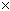SEARCH HOMEMath Central Quandaries & QueriesQuestion from Derek: Could you please tell me how to figure out the PST and GST paid on an item. Example: I paid $10 total (including taxes) and the GST is 6% and the PST is 7.5%, HOWEVER, in Quebec, we pay the PST on the sub-total+GST. Thanks, DerekHi Derek. Taxes can indeed be confusing in Canada. Take a look at this web page which summarizes this across Canada. You're right: in Quebec, GST is charged, then a subtotal is created that includes the GST and the PST is charged on that subtotal. You pay provincial sales tax on the federal tax. In your question, you said the final total including taxes was$10. So

$10 = s + 0.075s where s is the subtotal. So s =$10 / 1.075. Since 0.075s is the PST you paid, that
means

PST = 0.075 ($10 / 1.075) =$10 (0.06977) = $0.70 As well, s = c + 0.06c where c is the cost of the goods before any taxes. So c = s / 1.06. Since 0.06c is the GST you paid, this means: GST = 0.06 (s / 1.06) = 0.06 ( ($10 / 1.075) / 1.06) = $10 (0.05265) =$ 0.53

To summarize in general, if the final price is f and this includes GST and PST in Quebec:

PST = 0.06977 f
GST = 0.05265 f

Hope this helps,
Stephen La Rocque.

Derek wrote back:

I have a follow up question to this one previously posted:

Before July 1, 2006, the GST was 7%... pardon my ignorance, but how would I figure it out then?
Thanks so much!

Hi Derek.

In that case, PST is calculated the same way, but the factor for the GST calculation is

GST = 0.07 / (1.0751.07) f = 0.06086 f

Do you see how that is done?
Stephen La Rocque.

Question from Pierre:

In the solution above done by Stephen La Roque which is very useful I am wondering how he gets the 1.075 for pst and 1.06 for gst in the equation. Could please explain me how?

Hi Pierre,

In the line Stephen wrote

$10 = s + 0.075s = s + 0.075s think of s as 1s and then the line becomes$10 = 1s + 0.075s

At this point you can see s as a common factor so the expression can be written

\$10 = 1s + 0.075s = (1 + 0.075)s = 1.075s

I hope this helps,
HarleyMath Central is supported by the University of Regina and The Pacific Institute for the Mathematical Sciences.